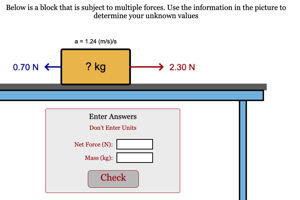Information About The Program
Newton's Law Simple (Homework)Students must calculate one of the parameters of a situation where Newton's Law applies to one object.
Below are any Resources that go with this program